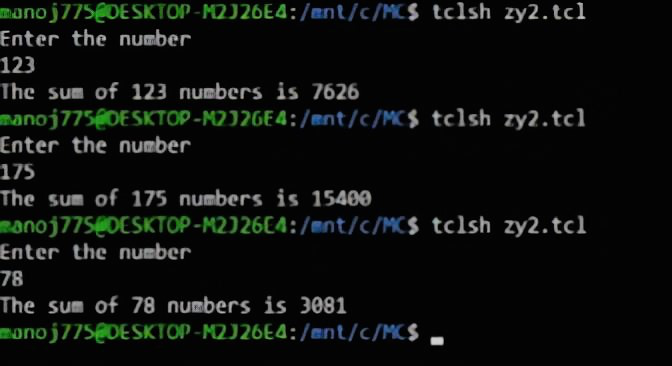Skip to content
Related Articles
TCL script to demonstrate recursive procedures
• Last Updated : 10 May, 2021

In this article, we will go through a unique set of TCL procedures that call themselves. These procedures as you might have already guessed are called recursive procedures. These are similar to recursive functions in other languages. Hence, to understand them even better, we will be considering the same procedure written as a recursive function in C programming to understand the syntax even better.

Pre-requisite –
If you want to know more about TCL, then kindly go through the following articles as follows.

Example –
We will consider a simple example where our objective is to find the sum of n natural numbers by calling sumofnnumbers {} recursively. Let’s understand this code in blocks.

Step-1 :
Our first step is to define a procedure that calls itself until n=1. We use the proc keyword to define a procedure. Before we do so let’s consider how a regular procedure would look like to find the sum of n natural numbers.

```proc sumofnnumbers {a} {
set sum 0
for {set i \$a} {\$i>=1} {incr i -1} {
set sum [expr \$sum+\$i]
}
return \$sum
}```

As you can see above we define the procedure using the conventional for-loop. And of course, we can either count from 1 to n or from n to 1. In this case, we have counted from n to 1.

Step-2 :
We can achieve the same procedure shown above with a recursive procedure that calls itself up to n=1 and achieves functionality similar to a conventional looping statement.

```proc sumofnnumbers {a} {
if {\$a>0} {
return [expr \$a+[sumofnnumbers [expr \$a-1]]]
} else {
return 0
}
}```

Note :

• The syntax of a procedure must be exactly as shown above. If you neglect the spaces or open the curly brace on a new line, the result will be an error.
• The same procedure can be used to find the product of n natural numbers. All you need to do is replace ‘+’ with ‘*’ and return 1 instead of 0 in the else block.

Step-3 :
Let’s compare the same block of code to what It would look like in C language to better understand the syntax.

```int sumofnnumbers(int a)
{
if(a>0)
{
return a+sumofnnumbers(a-1);
}
else
{
return 0;
}
}```

Step-4 :
The next step is to prompt the user to enter a number and pass it as an argument to the procedure – sumofnnumbers {}. We use gets to take input from the user.

```puts "Enter the number"
gets stdin a
puts "The sum of \$a numbers is [sumofnnumbers \$a]"```

Step-5 :
The whole code with the output is as follows.

Code –

```proc sumofnnumbers {a} {
if {\$a>0} {
return [expr \$a+[sumofnnumbers [expr \$a-1]]]
} else {
return 0
}
}
puts "Enter the number"
gets stdin a
puts "The sum of \$a numbers is [sumofnnumbers \$a]"```

Output :Attention reader! Don’t stop learning now. Get hold of all the important CS Theory concepts for SDE interviews with the CS Theory Course at a student-friendly price and become industry ready.

My Personal Notes arrow_drop_up